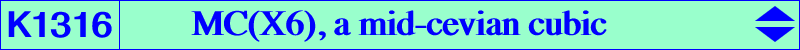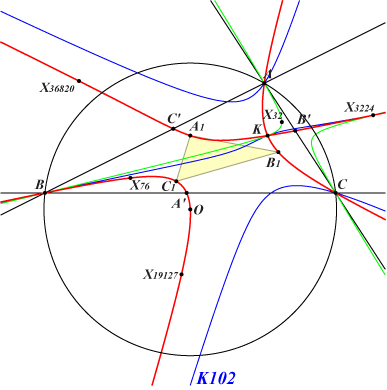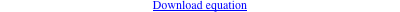too complicated to be written here. Click on the link to download a text file.X(3), X(6), X(76), X(599), X(3224), X(9462), X(14608), X(19127), X(36820), X(36821), X(36822), X(36823), X(36879), X(36880), X(36881), X(46023), X(46024) A1, B1, C1 vertices of the 1st Brocard triangle infinite points of K102 points of pK(X6,X3734) on (O) Geometric properties :K1316 is the mid-cevian cubic MC(X6) as in CL069. See also MC(X4) = K616. K1316 is a nodal cubic with node X(6). The nodal tangents are parallel to the asymptotes of the circum-conic with perspector X(3005), passing through X6, X39, X76, X141, X755, X882, X1843, X2353, etc. K1316 meets the sideline BC at A', on the line passing through A1 and X(19127). B' and C' are defined likewise. The isogonal transform of K1316 is K1317.